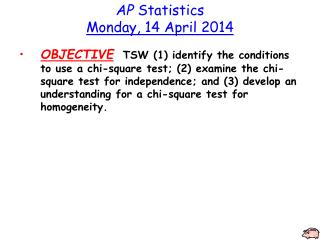DownloadDownload PresentationAP Statistics Monday, 14 April 2014

# AP Statistics Monday, 14 April 2014

Télécharger la présentation## AP Statistics Monday, 14 April 2014

- - - - - - - - - - - - - - - - - - - - - - - - - - - E N D - - - - - - - - - - - - - - - - - - - - - - - - - - -
##### Presentation Transcript

1. AP StatisticsMonday, 14 April 2014 • OBJECTIVETSW (1) identify the conditions to use a chi-square test; (2) examine the chi-square test for independence; and (3) develop an understanding for a chi-square test for homogeneity.

2. Chi-square testorc2 test

3. Suppose we are interested in seeing if some “fuzzy” dice are considered “fair”? What can we do?

4. Chi-square test • Used to test the counts of categorical data • Three types • Goodness of fit (univariate) • Independence (bivariate) • Homogeneity (univariate with two samples)

5. c2 distribution – df=3 df=5 df=10

6. c2distribution – • Different df have different curves • Skewed right • As df increases, curve shifts toward right & becomes more like a normal curve

7. c2 assumptions • SRS – reasonably random sample • Have countsof categorical data & we expect each category to happen at least once • Sample size – to insure that the sample size is large enough we should expect at least five in each category. ***Be sure to list expected counts!! Combine these together: All expected counts are at least 5.

8. c2 formula

9. c2 Goodness of fit test Based on df – df = number of categories - 1 • Uses univariate data • We want to see how well the observedcounts “fit” what we expect the counts to be • Use c2cdf function on the calculator to find p-values

10. Hypotheses – written in words H0: the observed counts equal the expected counts Ha: the observed counts are not equal to the expected counts Be sure to write in context!

11. Let’s test our dice! No, let’s skip this part.

12. Example 1 Does your zodiac sign determine how successful you will be? Fortune magazine collected the zodiac signs of 256 heads of the largest 400 companies. Is there sufficient evidence to claim that successful people are more likely to be born under some signs than others? Aries 23 Libra 18 Leo 20 Taurus 20 Scorpio 21 Virgo 19 Gemini 18 Sagittarius 19 Aquarius 24 Cancer 23 Capricorn 22 Pisces 29 How many would you expect in each sign if there were no difference between them? How many degrees of freedom? I would expect CEOs to be equally born under all signs. So 256/12 = 21.333333 Since there are 12 signs – df = 12 – 1 = 11

13. Assumptions: • Have a random sample of CEO’s • All expected counts are greater than 5. (I expect 21.33 CEO’s to be born in each sign.) • H0: The number of CEO’s born under each sign is the same. • Ha: The number of CEO’s born under each sign is different. • P-value = c2cdf(5.094, 10^99, 11) = 0.9265a= 0.05 • Since p-value > a, I fail to reject H0. There is not sufficient evidence to suggest that the number of CEOs born under each sign is different.

14. Example 2 A company says its premium mixture of nuts contains 10% Brazil nuts, 20% cashews, 20% almonds, 10% hazelnuts and 40% peanuts. You buy a large can and separate the nuts. Upon weighing them, you find there are 112 g Brazil nuts, 183 g of cashews, 207 g of almonds, 71 g or hazelnuts, and 446 g of peanuts. You wonder whether your mix is significantly different from what the company advertises. Why is the chi-square goodness-of-fit test NOT appropriate here? What might you do instead of weighing the nuts in order to use chi-square? Because we do NOT have counts of the type of nuts. We could count the number of each type of nut and then perform a c2 test.

15. Example 3 Offspring of certain fruit flies may have yellow or ebony bodies and normal wings or short wings. Genetic theory predicts that these traits will appear in the ratio 9:3:3:1 (yellow & normal, yellow & short, ebony & normal, ebony & short) A researcher checks 100 such flies and finds the distribution of traits to be 59, 20, 11, and 10, respectively. What are the expected counts? df? Are the results consistent with the theoretical distribution predicted by the genetic model? (see next page) Since there are 4 categories, df = 4 – 1 = 3 Expected counts: Y & N = 56.25 Y & S = 18.75 E & N = 18.75 E & S = 6.25 We expect 9/16 of the 100 flies to have yellow and normal wings. (Y & N)

16. Assumptions: • Have a random sample of fruit flies • All expected counts are greater than 5. • Expected counts: • Y & N = 56.25, Y & S = 18.75, E & N = 18.75, E & S = 6.25 • H0: The distribution of fruit flies is the same as the theoretical model. • Ha: The distribution of fruit flies is not the same as the theoretical model. • P-value = c2cdf(5.671, 10^99, 3) = 0.129 a = .05 • Since p-value > a, I fail to reject H0. There is not sufficient evidence to suggest that the distribution of fruit flies is not the same as the theoretical model.

17. Assignment • WS Chi Square #1 • Due on Thursday, 17 April 2014.